# jQuery: Selectors, Methods, and Traversing Through Examples

### In my first post, I introduced some of the basics in jQuery: selectors, the DOM, and how to traverse the DOM (by eq() and find() methods). In this post, I am going to dig deeper into selectors, methods, and traversing the DOM in jQuery while focusing on the practical aspects by offering examples.

· Web Dev Zone · Tutorial
Save
4.64K Views

## Introduction

In my first post, I introduced some of the basics in jQuery: selectors, the DOM, and how to traverse the DOM (by eq() and find() methods). In this post, I am going to dig deeper into selectors, methods, and traversing the DOM in jQuery while focusing on the practical aspects by offering examples, because I think that the most effective way to learn to program is by practicing.

## Background

Before heading on to the examples, I assume that you know the essential features of jQuery . They are very important if you want to become a 'jQuery guru'. The following are the essential features of jQuery:

• The HTML DOM

• jQuery Selectors

• jQuery Methods

• Traversing the DOM

• jQuery Ajax

• jQuery Effects

I have looked at many websites to learn jQuery, but I like the www.w3schools.com website best. You can visit it to learn (or review) anything that you want about jQuery (or other things).

Now, let's take a look at the two following examples to better understand selectors, jQuery methods, traversing the DOM, and how to apply them to real applications.

### Example1 – TryIt (Looks Like W3Schools)

Idea: When I was learning on the W3Schools website, I particularly appreciated the Try it yourself button. By clicking on this button, you will be navigated to the Tryit Editor where you can edit the code and view the result by clicking on the See Result button. It looks like this: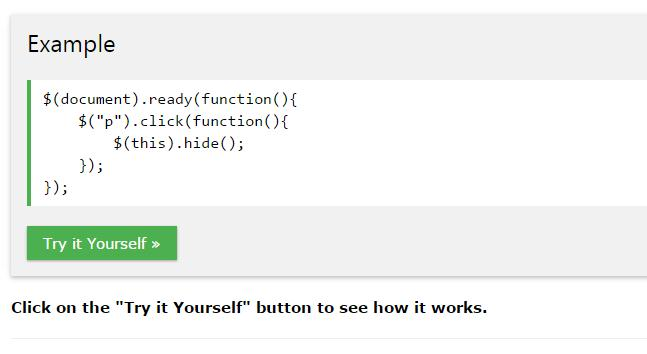And, the Tryit Editor looks like this: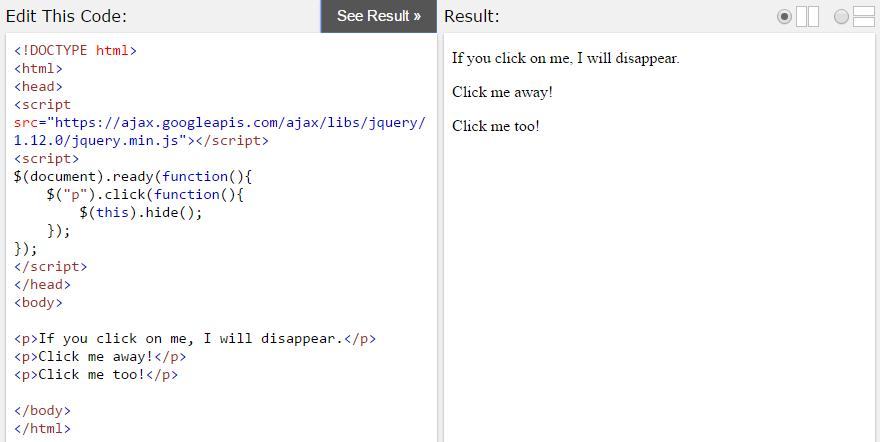From my observations, I created this Tryit (like Tryit Editor) example for someone, who is learning HTML. My Tryit looks like this: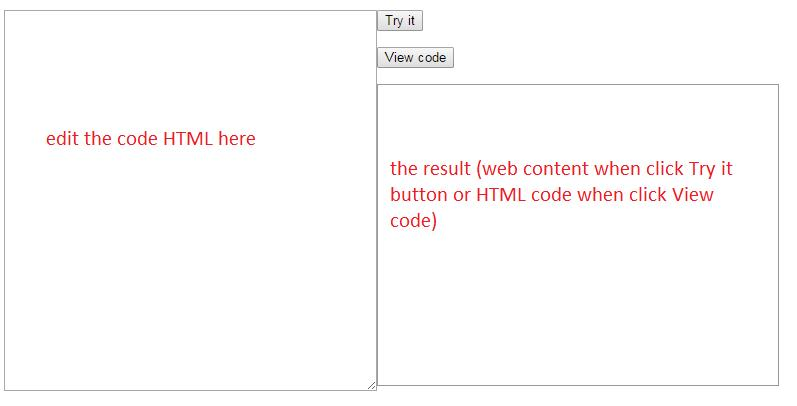And, what it looks like when running a sample: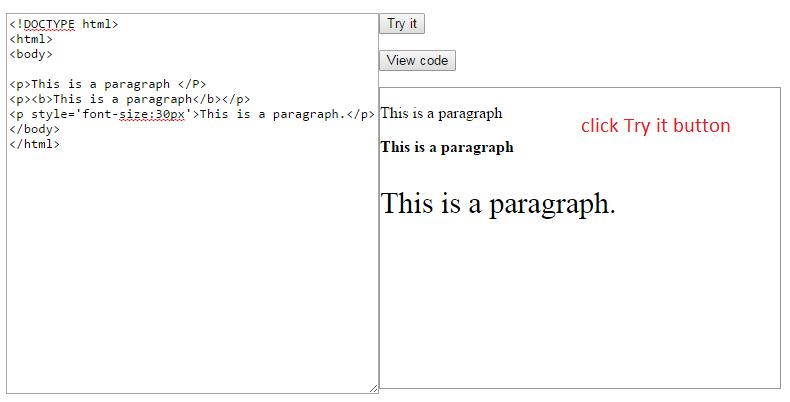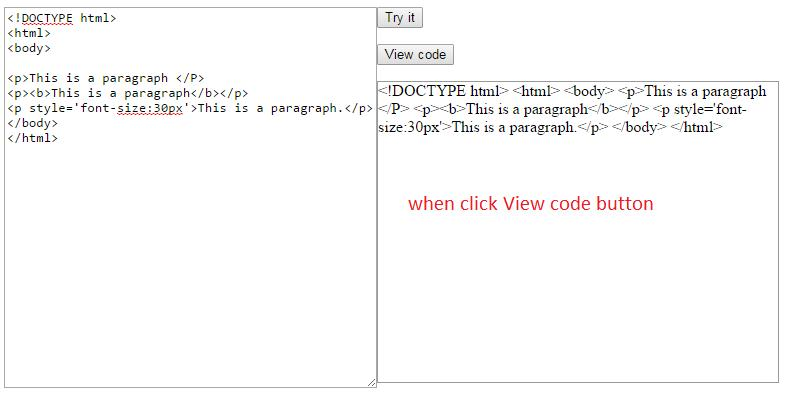Looks great! But my Tryit only uses HTML markup, not useful for scripts (jQuery, JavaScript, etc). You can write some code to complete it (hopefully).

Code: To create the Tryit example, you need to prepare some HTML markup (in your HTML file) and some jQuery code (in your JS file).

HTML Markup

``````<BODY>
<div id="container">
<div id="input">
<textarea id="message" rows="25" cols="50"></textarea>
</div>
<p><button id ='tryit' >Try it</button></p>
<p><button id = 'code'>View code</button></p>
<div id="output">
</div>
</div>
</BODY>``````

Some Styles in CSS file

``````#input{float:left;}
#output{height:300px; width:400px;border:1px #999 solid;float:left;}
#container{width:950px;}``````

jQuery code

``````\$(document).ready(function(){
//code for the Try it button
\$('#tryit').click(function(){
//using parseHTML method to convert the string (in textarea)
//to a set of DOM nodes
var html = \$.parseHTML(\$('#message').val());
//setting the above content to the #output element
\$('#output').html(html);
});
//code for the View code button
\$('#code').click(function(){
//setting the text content of the textarea to #output
\$('#output').text(\$('#message').val());
});
});``````
• parseHTML():  converts  the string to a set of DOM nodes.

• html(): sets or returns the content of the selected elements.

• text(): sets or returns the text content of the selected elements.

•  val(): returns or sets the value attribute of the selected elements.

### Example2 – CRUD (Looks Like CRUD Applications)

Idea: When I was learning the visual programming languages (VB.NET, C#, etc), in the database programming part there's a classic application, the CRUD (Create, Read, Update, Delete) application, which looks like:I suddenly thought that I could create an example that looks like a CRUD application by using HTML and jQuery, of course.

Code: I am going to represent my example step by step, and I hope you can easily follow it.

Step 1: First, we create a data table that looks like this: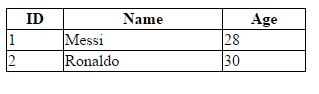HTML Markup

``````<table>
<tr>
<th>ID</th>
<th>Name</th>
<th>Age</th>
</tr>
<tr>
<td>1</td>
<td>Messi</td>
<td>28</td>
</tr>
<tr>
<td>2</td>
<td>Ronaldo</td>
<td>30</td>
</tr>
</table>``````

Some Styles

``````table {width:300px; margin-bottom:20px;border-collapse:collapse; }
tr,th, td {border: 1px black solid;}``````

If you have knowledge about relational databases, then in our data table, the ID column looks like a primary key, which uniquely identifies each row (record) in a database table (our data table also).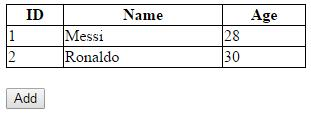HTML Markup

``<button id = 'add' >Add</button>``

When I click the Add button I want it to display the form with three fields: ID, Name, and Age. And, we will fill the information into these fields and insert them to the table. Here's what it looks like when I click the Add button: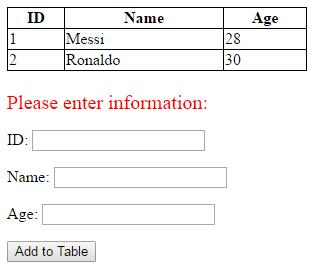Look at the above figure, we need to add the form (with three textboxes), the Please enter information text line, and the Add to Table button (They are hidden before I click the Add button.)

Full HTML Markup

``````<table>
<tr>
<th>ID</th>
<th>Name</th>
<th>Age</th>
</tr>
<tr>
<td>1</td>
<td>Messi</td>
<td>28</td>
</tr>
<tr>
<td>2</td>
<td>Ronaldo</td>
<td>30</td>
</tr>
</table>
<form>
<p>ID: <input id ='id' type = 'text'/></p>
<p>Name: <input id = 'name' type = 'text'/></p>
<p>Age: <input id ='age' type = 'text'/></p>
</form>

Some styles for the Please enter information text line:

``#info {color:red; font-size:20px;}``

jQuery Code

We will hide the form, the Please enter information text line, and the Add to Table button before the Add button is clicked. Some code here:

``````\$(document).ready(function(){
\$('form').hide();//hide the form
});``````

When I click the Add button, these components will be displayed to enter information. Some code in the click event of the Add button:

``````\$('#add').click(function(){
\$('form').show();// show the form
\$(this).hide();// hide the first 'Add' button
});``````

Next, I will enter information into text boxes and click Add to Table to insert them to my table, it looks like: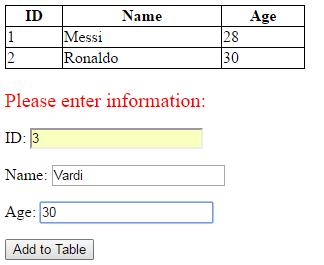When you click Add to Table: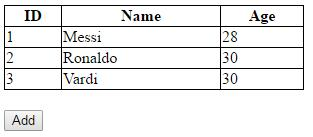We also need to acknowledge some special cases such as: ID must be NOT NULL and not allow the user to enter an ID that already exists in the table. It looks like: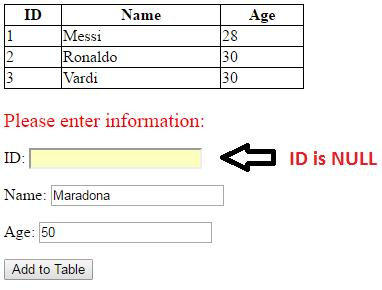Or: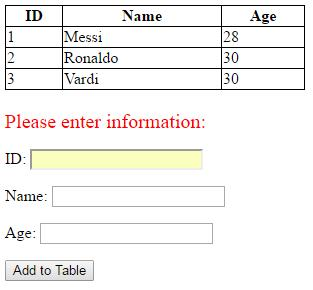Or: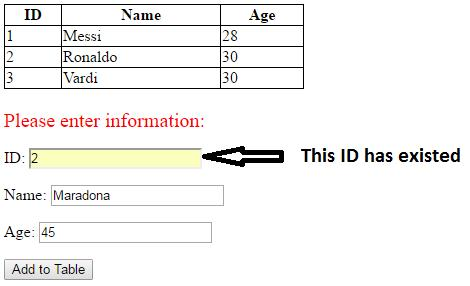In all of the above special cases, when I click the Add to Table button, it will display the notification like this:And, the jQuery code for the click event of the Add to Table button:

``````\$('#addform').click(function(){
var s ='';
//gets value from the ID textbox
var id =\$('#id').val();
//gets value from the Name textbox
var name = \$('#name').val();
//gets value from the Age textbox
var age =\$('#age').val();
//loops through all cells at the ID column
for(var i=0; i<= \$('td').length; i=i+3)
//checks ID has existed in the Table or ID is NULL
if(\$("td").eq(i).text() == id || id =="")
{
\$('form').hide();
\$('#info').hide();
return false;//break
}
//saving information of the new row
s = s + '<tr>' + '<td>' + id + '</td>' + '<td>' + name
+ '</td>' + '<td>' + age + '</td>' + '</tr>';
\$('form').hide();
\$('#info').hide();
//insert the new row to the table by the insertAfter method
\$(s).insertAfter(\$("table tr:last"));
});``````

And, we also need to attend to the final case... that is, when I insert a new row into the table then next time when I click the ‘Add’ button again to insert another new row, we can see information which we have just entered before still exist. It looks like this: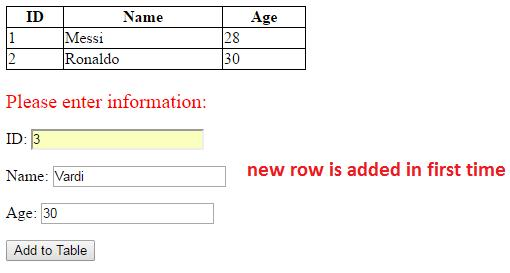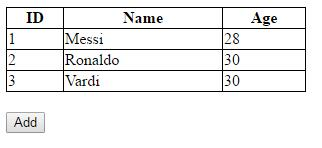When I click the Add button again, you can see this: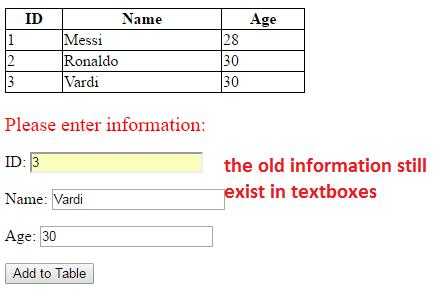I want the textboxes to be emptied, so I have created a function outside my document ready event. This function name is refresh and is defined as follows:

``````\$(document).ready(function(){
//….
});
function refresh()
{
\$('#id').val("");
\$('#name').val("");
\$('#age').val("");
}``````

And, this function is called in the click event of the Add button like follows:

``````\$('#add').click(function()
{
\$('form').show();
\$('#info').show();
refresh();// call refresh function to empty textboxes
\$(this).hide();
});``````

Looks great! We have completed the Add button. Now, we’re going to move on to the next step.

Step 2: I add the Delete button, whic looks like: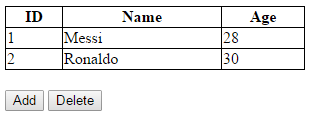When I click this button, I want a textbox to be displayed as follows: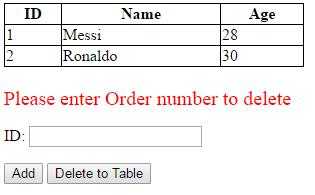If you enter an ID which already exist in Table (such as, 1 or 2) and click the Delete to Table button then the row with the corresponding ID will be deleted, likes this: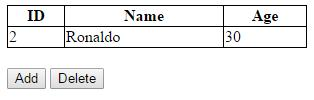If you enter an ID that doesn’t exist in the table, a message will be displayed: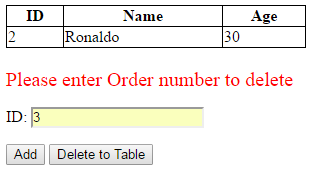We can use the form in Step 1 but hide the Name textbox and Age textbox, and we also use the refresh() function again to empty the ID textbox. Code here:

HTML Markup

``````<button id = 'delete' >Delete </button>
<button id = 'deleteform'>Delete to Table</button>``````

jQuery Code

``````\$('#deleteform').hide();
\$('#delete').click(function(){
\$('form').show();
\$('#info').show();
refresh();//call refresh() function
//to delete' by text() method
\$('#info').text('Please enter Order number  to delete');
\$('form p').eq(1).hide();// hide Name textbox
\$('form p').eq(2).hide();// hide Age textbox
\$(this).hide();// hide the ‘Delete’ button
\$('#deleteform').show();//show the ‘Delete to Table’ button
});
\$('#deleteform').click(function(){
var id = \$('#id').val();
\$('form').hide();
\$('#info').hide();
\$('#deleteform').hide();
\$('#delete').show();
for(var i=0; i<= \$('td').length; i=i+3)
if(\$("td").eq(i).text() == id)// check ID already exist in Table
{
\$("td").eq(i).next().next().remove();// delete Age cell  of row
\$("td").eq(i).next().remove();//delete Name cell of row
\$("td").eq(i).remove();//delete ID cell of roww
return false;//break
}
//If  ID doesn’t exist in the Table, a message will display
\$('#info').hide();
\$('form').hide();
\$('#deleteform').hide();
\$('#delete').show();
});``````

Now we are going to go to Step 3.

Step 3: I add the Modify button. This button’s function is to change the information of a particular row in the table base on its ID. You can see some of the results: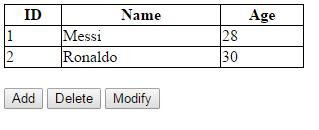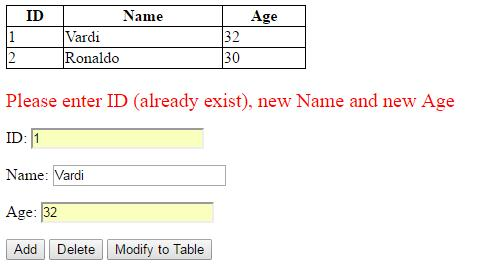If ID doesn’t exist in the table, a message will display: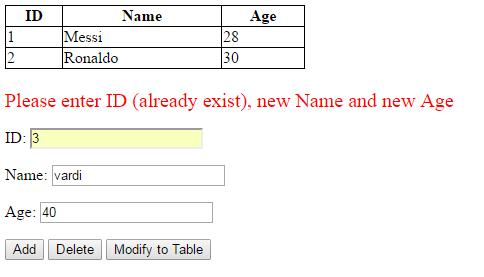HTML Markup

``````<button id = 'modify' >Modify</button>
<button id= 'modifyform'>Modify to Table</button>``````

jQuery Code

``````\$('#modifyform').hide();
\$('#modify').click(function(){
\$('form').show();
\$('#info').show();
refresh();
\$('form p').eq(1).show(); //show Name textbox
\$('form p').eq(2).show();// show Age textbox
\$(this).hide();
\$('#modifyform').show();
});
\$('#modifyform').click(function(){
var id =\$('#id').val();
var name = \$('#name').val();
var age =\$('#age').val();
\$('form').hide();
\$('#info').hide();
\$('#modifyform').hide();
\$('#modify').show();
for(var i=0 ; i<= \$('td').length; i=i+3)
if(\$("td").eq(i).text() == id)
{
if (age != '')
\$("td").eq(i).next().next().text(age);//modify Age value
if(name != '')
\$("td").eq(i).next().text(name);//modify Name value
return false;//break
}
// if ID doesn’t exist
\$('form').hide();
\$('#info').hide();
\$('#modifyform').hide();
\$('#modify').show();
});``````

Step 4: Now, I want to navigate through the rows of the table. I am going to add the following buttons:

• The Next button: navigate to next row from a particular row

• The Prev button: navigate to previous row from a particular row

• The First button: navigate to the first row

• The Last button: navigate to the last row

Result is Displayed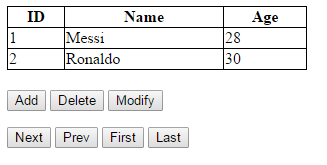HTML Markup

``````<button id ='next' >Next</button>
<button id ='prev'>Prev</button>
<button id ='first'>First</button>
<button id ='last'>Last</button>``````

jQuery Code

``````\$(document).ready(function(){
var pos = 1;// global variable
//next button
\$('#next').click(function(){
// check if not the last row
if (pos < \$('tr').length)
{
pos = pos + 1;//move to next position
\$('tr').eq(pos-1).css('background','none');
//highlight the row at the current pos
\$('tr').eq(pos).css('background','#666');
}
});
//prev button
\$('#prev').click(function(){
//check if not the first row
if (pos > 0)
{
pos = pos - 1;// move to previous position
//highlight the row at the current pos
\$('tr').eq(pos).css('background','#666');
\$('tr').eq(pos+1).css('background','none');
}
\$('tr').eq(0).css('background','none');
});
//first button
\$('#first').click(function(){
\$('tr').css('background','none');
pos = 1;//position = 1
\$('tr').eq(1).css('background','#666');//highlight the first row
});
//last button
\$('#last').click(function(){
\$('tr').css('background','none');
pos = \$('tr').length-1; // the position of the last row
\$('tr:last').css('background','#666');//highlight the last row
});
});``````

Now we're going to go to the final step.

Final Step (Finish)

Assume that I have a situation as follows: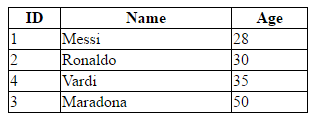We can see the above table, ID is unordered. Suppose I want to be able sort the rows of the table in ascending order or descending order based on the ID value. So, I have created two buttons as follows:

• The Asc Order Sort button: sort the rows in ascending order base on ID

• The Desc Order Sort button: sort the rows in descending order base on ID

HTML Markup

``````<button id = 'sortasc'>Asc Order Sort</button>
<button id ='sortdesc'>Desc Order Sort</button>``````

jQuery Code

``````\$('#sortasc').click(function(){
\$('form').hide();
var id =""; //local temporary variable
var name ="";//local temporary variable
var age = "";//local temporary variable
for(var i=0 ; i<= \$('td').length - 1; i=i+3)
//loop through all cells at the ID column
//check if value at ith cell > value at jth cell (with i<j)
//then swap cells of the ith row and cells of the
//jth row or value at cells = NULL
if(\$("td").eq(i).text()!=''&& \$("td").eq(i+3).text()!=''&&
\$("td").eq(i).text() > \$("td").eq(i+3).text())
{
//swap the ID cells
id = \$("td").eq(i).text();
\$("td").eq(i).text(\$("td").eq(i+3).text());
\$("td").eq(i+3).text(id);
//swap the Name cells
name = \$("td").eq(i).next().text();
\$("td").eq(i).next().text(\$("td").eq(i+3).next().text());
\$("td").eq(i+3).next().text(name);
//swap the Age cells
age = \$("td").eq(i).next().next().text();
\$("td").eq(i).next().next().text(\$("td").eq(i+3).next().next().text());
\$("td").eq(i+3).next().next().text(age);
// trigger click event automatically
\$('#sortasc').trigger("click");
}
});
\$('#sortdesc').click(function(){
\$('form').hide();
var id ="";//local temporary variable
var name ="";//local temporary variable
var age = "";//local temporary variable
for(var i=0; i<= \$('td').length - 1; i=i+3)
//loop through all cells at the ID column
//check if value at ith cell < value at jth cell (with i<j) then swap
//cells of the ith row and cells of the jth row or value at cells = NULL
if(\$("td").eq(i).text()!=''&& \$("td").eq(i+3).text()!=''
&& \$("td").eq(i).text() < \$("td").eq(i+3).text())
{
//swap the ID cells
id = \$("td").eq(i).text();
\$("td").eq(i).text(\$("td").eq(i+3).text());
\$("td").eq(i+3).text(id);
//swap the Name cells
name = \$("td").eq(i).next().text();
\$("td").eq(i).next().text(\$("td").eq(i+3).next().text());
\$("td").eq(i+3).next().text(name);
//swap the Age cells
age = \$("td").eq(i).next().next().text();
\$("td").eq(i).next().next().text(\$("td").eq(i+3).next().next().text());
\$("td").eq(i+3).next().next().text(age);
// trigger click event automatically
\$('#sortdesc').trigger("click");
}
});``````

Final result: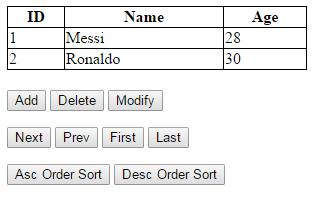Add some new rows into the table by using the Add button:Click the Asc Order Sort: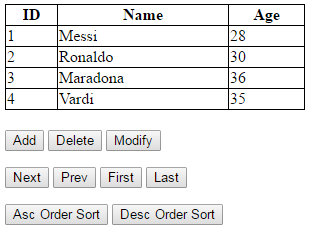Click the Desc Order Sort: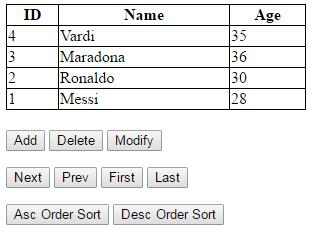Click the First button: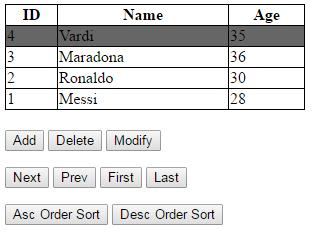Click the Last button:You can navigate through the rows by the Next and Prev buttons.

## Conclusion

In this article, I have introduced some features of jQuery: selectors, methods, and how to traverse the DOM by methods as next(), eq(), etc. In my next article, I will introduce some other features of jQuery such as effects, ajax, etc. And of course, I will focus on the practical aspect by providing examples. I hope you find my article helpful!

Topics:
web developement, jquery, dom, tutorial

Opinions expressed by DZone contributors are their own.